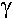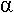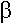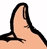# What's a Neper?

Click here to go to our page on calculating transmission line losses

The Neper (Np) is similar to the decibel (dB). It's unitless and is used to express ratios. The Neper uses the natural logarithm, and is named after Microwave Hall-of-Famer John Napier. Instead of using the logarithm function (base 10), the Neper uses the base "e". For voltage ratio of a/b, the difference in calculation is shown here:

dB=20*log(a/b)

Np=ln(a/b)

The difference between a dB and a Neper is just a fixed ratio. This is computed by dividing the two equations:

dB/Np=20*log(a/b)/ln(a/b) = 20/ln(10)=8.68588

The simplification is done by noting that ln(x)=log(x)*ln(10), if you remember your high-school math. Nepers are typically used in loss calculations in the attenuation constant of the propagation constant:=+ jwhereis in nepers/meter.

This is important, so remember it!How about we immortalize the Neper conversion as Microwaves101 Rule of Thumb #119:

To convert Nepers to decibels, multiply by (approximately) 8.69.

More accurately,

dB/Np = 20/ln(10)=8.68588

Author : Unknown Editor